# QOTD - Quantitative Aptitude - HCF & LCM Problem

2019-09-26 | Team PendulumEdu

The HCF of $$\frac{2}{25}$$,$$\frac{6}{5}$$, and $$\frac{8}{15}$$ will be

Options: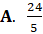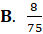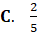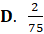Solution:

HCF of any fraction is given as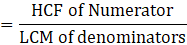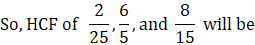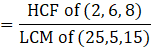HCF of two or more numbers is the greatest number which divides each of them exactly.

Thus, numbers which can exactly divide all the numbers 2, 6 and 8 will be either 1 or 2

Greatest number which divides each of them exactly will be 2

Thus, HCF of (2, 6 and 8) will be 2

LCM is the smallest number which is the multiple of all the numbers.

Thus, LCM of the numbers 25, 5, and 15 will be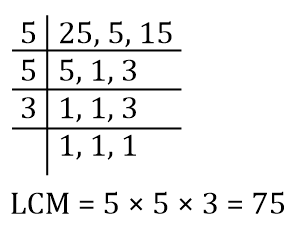Therefore,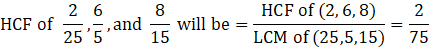Hence, (D) is the correct answer.

Such type of question is asked in Quantitative Aptitude/Numerical Ability section of various exams like SSC CGL, SSC MTS, SSC CPO, SSC CHSL, RRB JE, RRB NTPC, RRB GROUP D, RRB Office Assistant, IBPS Clerk, RBI Assistant, etc. Try and attempt free mock tests at PendulumEdu.0